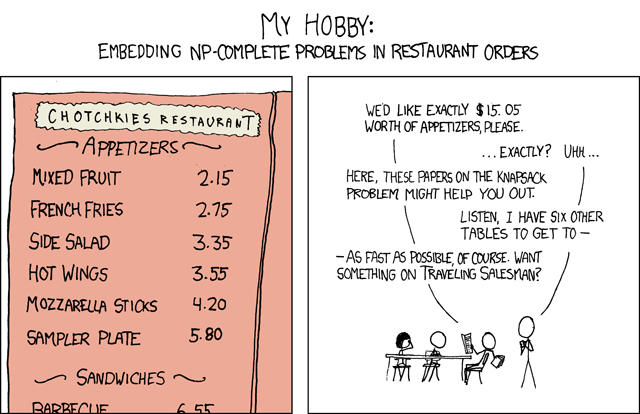# How would you input xkcd.com/287 into WolframAlpha?

In this XKCD comic, a stick figure asks an NP-complete problem to order exactly 15.05 worth of appetizers out of a menu that includes the following list of prices: {2.15, 2.75, 3.35, 3.55, 4.20, 5.80}.

What is the mathematical name and procedure for this kind of problem, and how would you input something like this to WolframAlpha if possible?• en.wikipedia.org/wiki/Knapsack_problem – Myself Jan 7 '12 at 14:01
• I think this question is ok. Please consider this comment a vote against closing it. – Srivatsan Jan 7 '12 at 14:27
• i couldnt get the joke in the xkcd link apparently :( – Bhargav Jan 7 '12 at 14:36
• Rather unfortunately, .01*IntegerPartitions[1505, All, {215, 275, 335, 355, 420, 580}] doesn't work in Alpha. – J. M. is a poor mathematician Jan 7 '12 at 16:01
• The stick figure poses an instance of an NP-complete problem. NP-completeness is a property of problems (which in complexity theory is synonymous with classes of problems), not of problem instances. For every problem instance, there's a trivial polynomial-time algorithm to solve it, namely the one that simply prints the solution. – joriki Jan 7 '12 at 16:07

To count the number of solutions, enter series for 1/((1-x^(215/100))(1-x^(275/100))(1-x^(335/100))(1-x^(355/100))(1-x^(420/100))(1-x^(580/100))) at x=0 (or click here), then press "More Terms" about two dozen times until you get the coefficient for $x^{1505/100}=x^{301/20}$, which is $2$, so there are two solutions.
For a slightly smaller problem, you could also get the solutions themselves by entering series for 1/((1-a x^(215/100))(1-b x^(275/100))(1-c x^(335/100))(1-d x^(355/100))(1-e x^(420/100))(1-f x^(580/100))) at x=0 (or clicking here) and decoding the coefficient of $x^{1505/100}$. Unfortunately Wolfram|Alpha stops offering more terms at $x^{10}$, so the best we can do is to deduce from the coefficient $a^3d+ef$ of $x^{10}$ that there are two appetizer combinations for exactly $\$10$, namely$3$mixed fruit and hot wings, or Mozzarella sticks and a sampler plate. To see why this works, you can read up on the generating function for the partition function. • SAGE says$(a^7+ad^2f)$for 15$05 – Myself Jan 7 '12 at 19:36
• @Myself - Nice. In alternative notation, $7a = 15.05$ and $a+2d+f=15.05$, where the letters label the appetizer prices in the order they're given. – r.e.s. Jan 8 '12 at 2:03